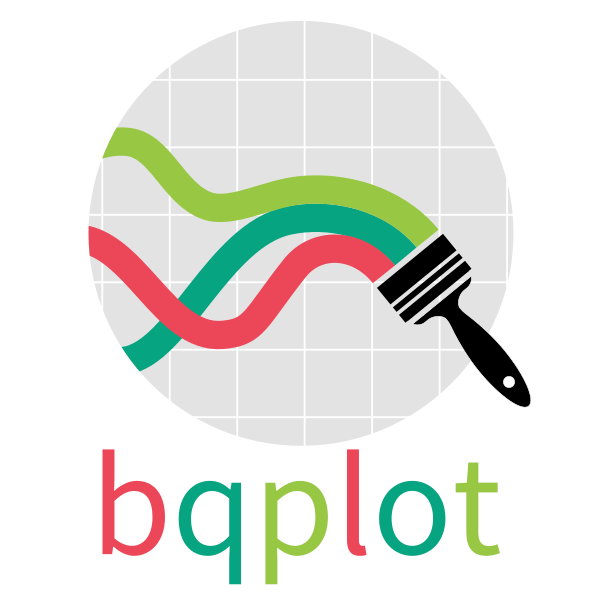# bqplot: An Interactive Plotting Library for the Jupyter NotebookImage credit: bqplot

## bqplot

Most of my open source work focuses on bqplot, an interactive visualization library for the Jupyter notebook. bqplot is based on the ipywidgets infrastructure, which I also used to work a great deal with.

One of the things (looking back at it) that I like best about bqplot is the object model API. It seems like a much more natural way to generate plots than some of the other Grammar of Graphics adaptations that I’ve seen. Partly I think because we were constantly shifting between application/widget development and library development - we represented users much better than other projects I’ve worked on.

For e.g. to generate a simple interactive scatter plot, first we import the requisite libraries:

import numpy as np

size = 100
np.random.seed(0)
x_data = np.arange(size)
y_data = np.cumsum(np.random.randn(size) * 100.0)

Then we import and declare the Scales, which are mappings from data to pixels.

from bqplot import LinearScale

# Let's create a scale for the x attribute, and a scale for the y attribute
x_sc = LinearScale()
y_sc = LinearScale()

Now we need to create the actual visualization (what we call the Mark). The Scatter object takes in the x_data, the y_data and maps them to the passed scales like below:

from bqplot import Scatter

# Let's create a scale for the x attribute, and a scale for the y attribute
scatter_chart = Scatter(x=x_data, y=y_data, scales={"x": x_sc, "y": y_sc})

We also need to draw Axis (which is the 1D visual representation of the Scale). Notice that all that separates the x-axis from the y-axis is the orientation parameter.

from bqplot import Axis

# Let's create a scale for the x attribute, and a scale for the y attribute
x_ax = Axis(label="X", scale=x_sc)
y_ax = Axis(label="Y", scale=y_sc, orientation="vertical")

Finally, we put it all together into a Figure, which we then display:

from bqplot import Figure
from IPython.display import display

fig = Figure(marks=[scatter_chart], title="A Figure", axes=[x_ax, y_ax])
display(fig)

The coolest part about bqplot is that this Figure is a live-object (an ipywidget), and so is every component of it! So, we can change it!

scatter_chart.y = 2 * y_data

You should see something like this!

We can make the plot interactive by allowing it to be moved.

scatter_chart.enable_move = True

Go ahead, drag one of the points!

Now, here’s where the magic of widgets comes in. Let’s create a function foo which will be called whenever the y-data of the scatter chart is changed.

def foo(change):
print("This function is called whenever the y-data is changed!")

Finally, we tell scatter_chart to call foo whenever y is changed. We do this by using observe to register the callback.

scatter_chart.observe(foo, 'y')

If you move any of the scatter_chart points, foo should be called! That’s it! That’s all it takes to make an interactive plot in bqplot.

## bqplot Talks

Here’s a video of us speaking about it at Scipy 2017!

I gave a more extended talk at PyData Seattle

And at and PyData Ann Arbor

For some of our Deep Learning visualizations, have a look at my talks at PyGotham

And at PyData NYC

## Licensing Information

This software was released under the Apache 2.0 license.